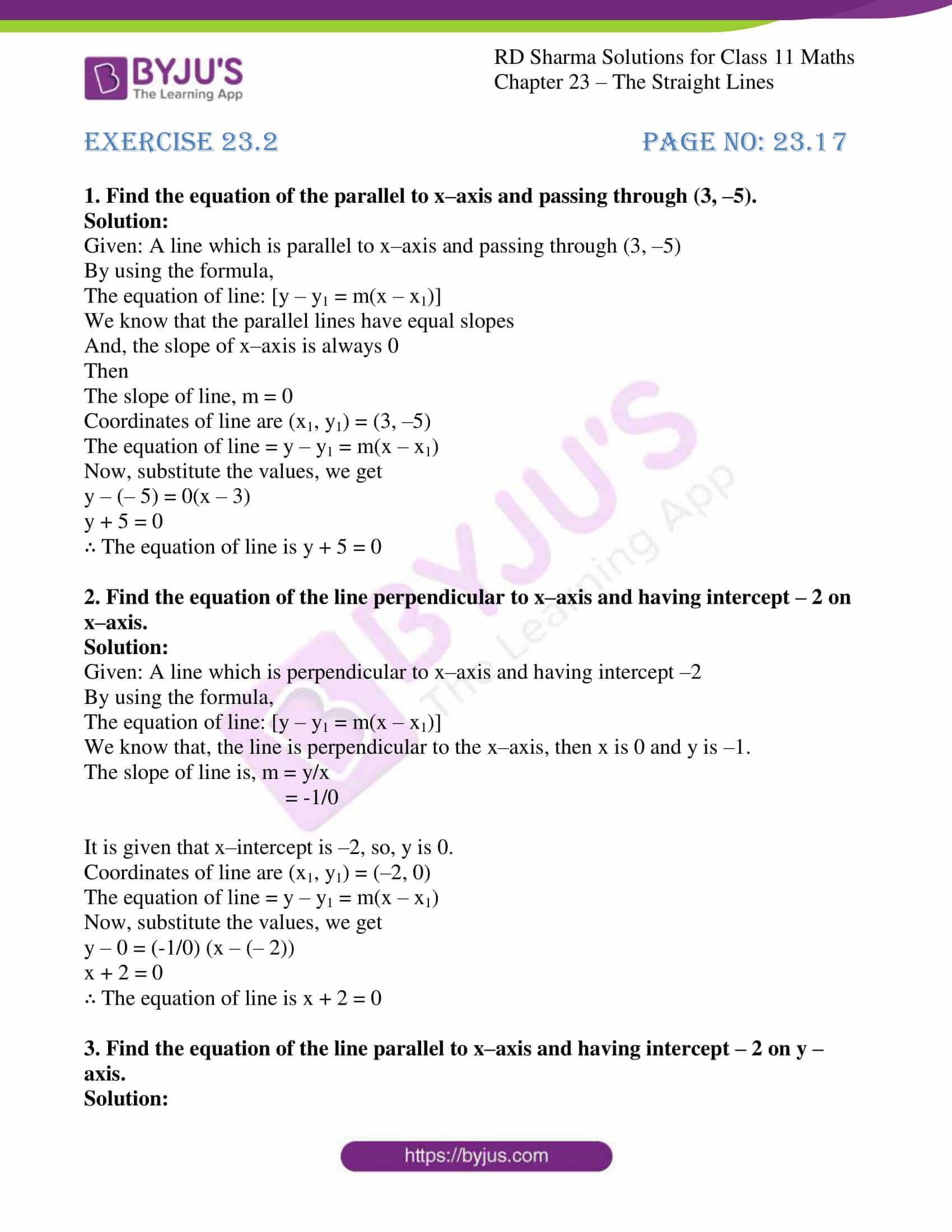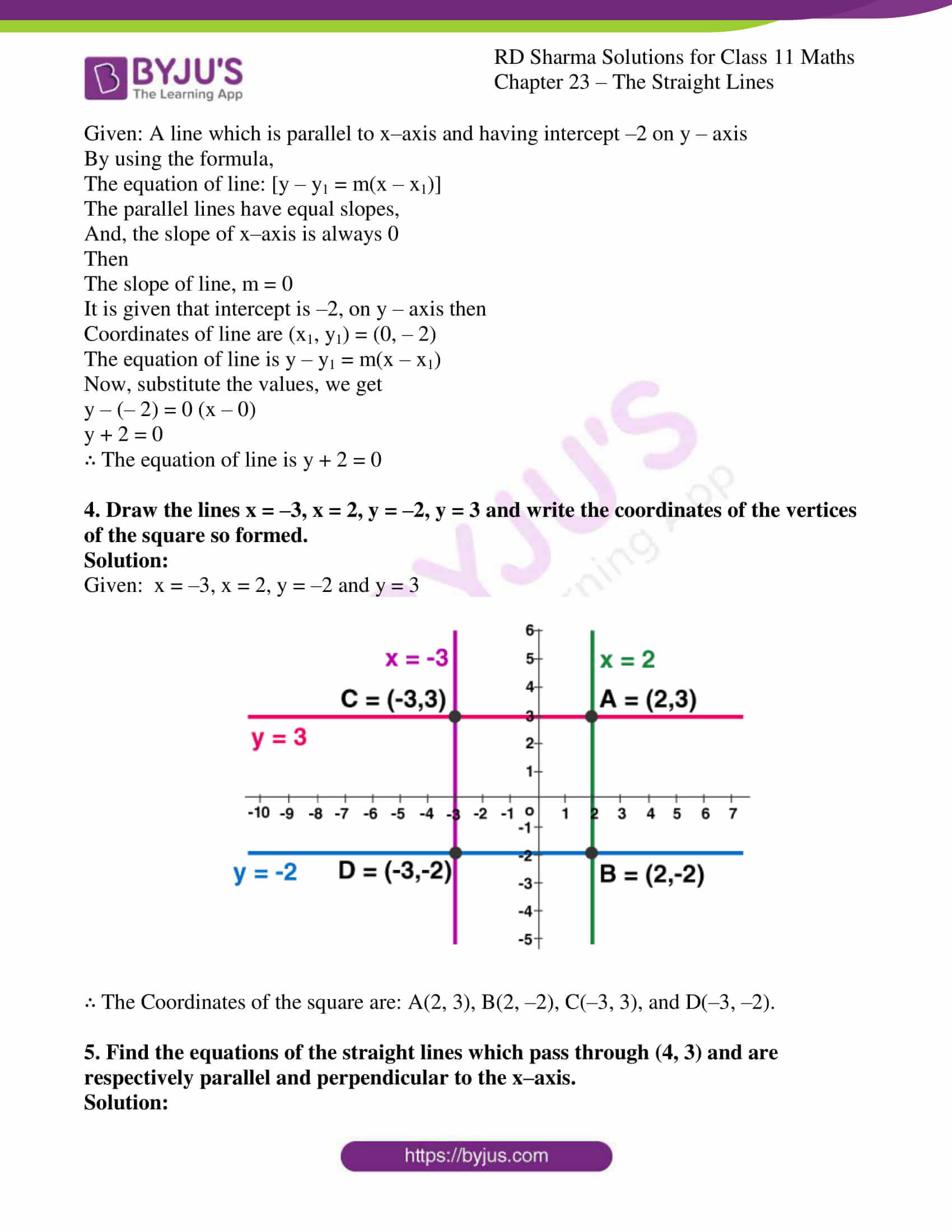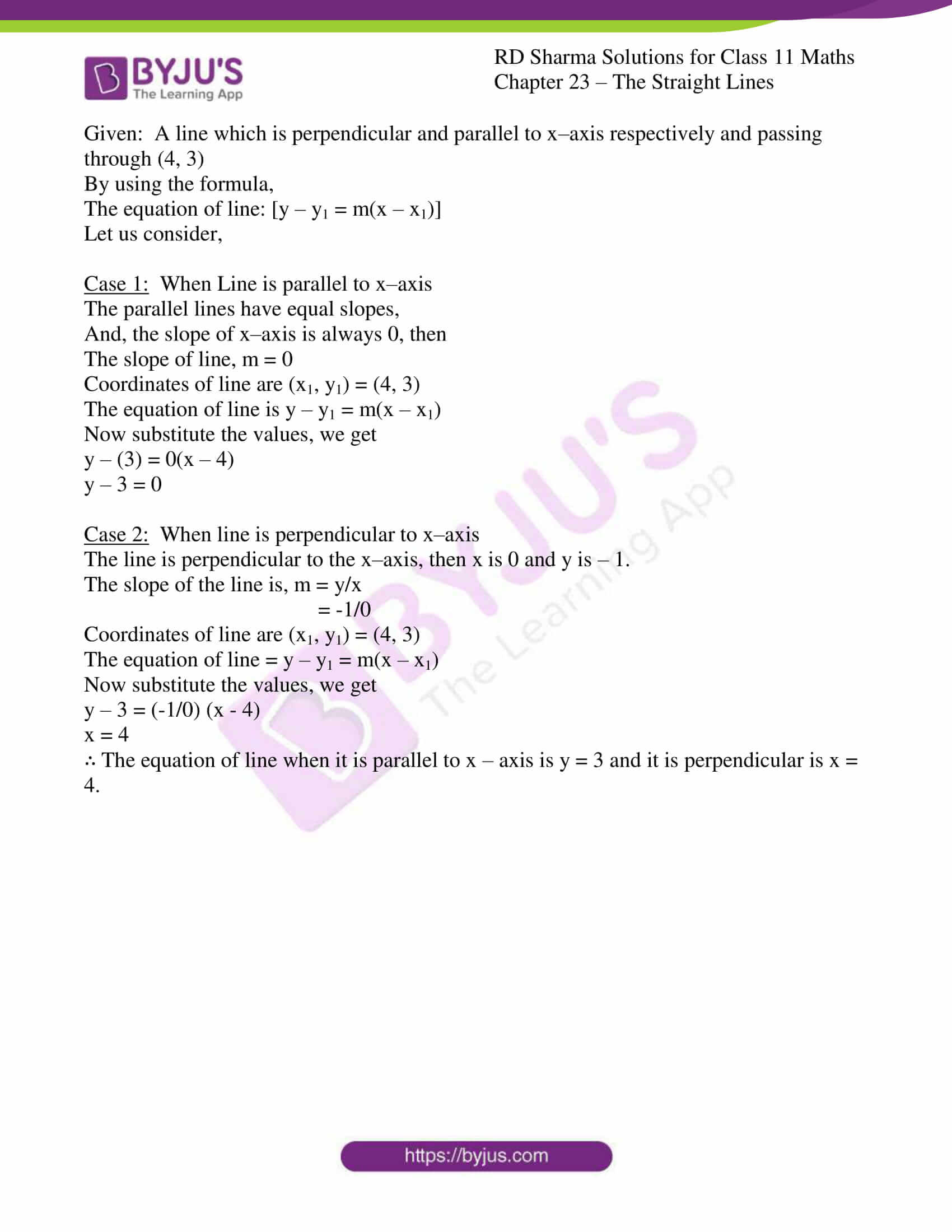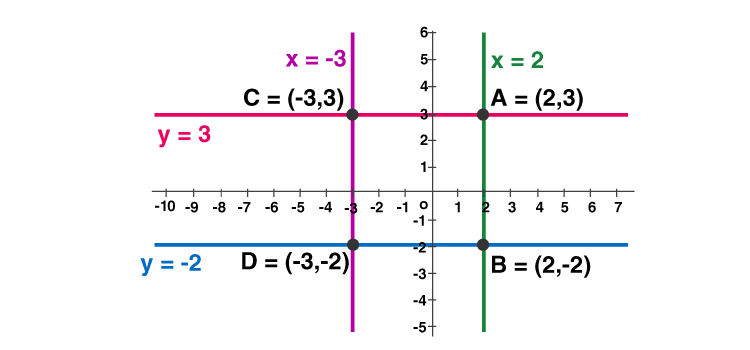# RD Sharma Solutions for Class 11 Chapter 23 - The Straight Lines Exercise 23.2

This exercise discusses the concepts related to intercepts of a line on the axes and equations of lines parallel to the coordinate axes, with suitable examples for better understanding. The problems present in the RD Sharma textbook are solved by experts at BYJU’S in a simple manner, depending upon the students’ grasping abilities. It is very important for the students to get well versed with these solutions to get a good score in the Class 11 examination. These solutions will help you understand and master different types of questions on straight lines. The pdf of RD Sharma Class 11 Maths Solutions is provided here. Students can refer and download from the given links.

## Download the pdf of RD Sharma Solutions for Class 11 Maths Exercise 23.2 Chapter 23 – The Straight Lines### Also, access other exercises of RD Sharma Solutions for Class 11 Maths Chapter 23 – The Straight Lines

Exercise 23.1 Solutions

Exercise 23.3 Solutions

Exercise 23.4 Solutions

Exercise 23.5 Solutions

Exercise 23.6 Solutions

Exercise 23.7 Solutions

Exercise 23.8 Solutions

Exercise 23.9 Solutions

Exercise 23.10 Solutions

Exercise 23.11 Solutions

Exercise 23.12 Solutions

Exercise 23.13 Solutions

Exercise 23.14 Solutions

Exercise 23.15 Solutions

Exercise 23.16 Solutions

Exercise 23.17 Solutions

Exercise 23.18 Solutions

Exercise 23.19 Solutions

### Access answers to RD Sharma Solutions for Class 11 Maths Exercise 23.2 Chapter 23 – The Straight Lines

#### EXERCISE 23.2 PAGE NO: 23.17

1. Find the equation of the parallel to x–axis and passing through (3, –5).

Solution:

Given: A line which is parallel to x–axis and passing through (3, –5)

By using the formula,

The equation of line: [y – y1 = m(x – x1)]

We know that the parallel lines have equal slopes

And, the slope of x–axis is always 0

Then

The slope of line, m = 0

Coordinates of line are (x1, y1) = (3, –5)

The equation of line = y – y1 = m(x – x1)

Now, substitute the values, we get

y – (– 5) = 0(x – 3)

y + 5 = 0

∴ The equation of line is y + 5 = 0

2. Find the equation of the line perpendicular to x–axis and having intercept – 2 on x–axis.

Solution:

Given: A line which is perpendicular to x–axis and having intercept –2

By using the formula,

The equation of line: [y – y1 = m(x – x1)]

We know that, the line is perpendicular to the x–axis, then x is 0 and y is –1.

The slope of line is, m = y/x

= -1/0

It is given that x–intercept is –2, so, y is 0.

Coordinates of line are (x1, y1) = (–2, 0)

The equation of line = y – y1 = m(x – x1)

Now, substitute the values, we get

y – 0 = (-1/0) (x – (– 2))

x + 2 = 0

∴ The equation of line is x + 2 = 0

3. Find the equation of the line parallel to x–axis and having intercept – 2 on y – axis.

Solution:

Given: A line which is parallel to x–axis and having intercept –2 on y – axis

By using the formula,

The equation of line: [y – y1 = m(x – x1)]

The parallel lines have equal slopes,

And, the slope of x–axis is always 0

Then

The slope of line, m = 0

It is given that intercept is –2, on y – axis then

Coordinates of line are (x1, y1) = (0, – 2)

The equation of line is y – y1 = m(x – x1)

Now, substitute the values, we get

y – (– 2) = 0 (x – 0)

y + 2 = 0

∴ The equation of line is y + 2 = 0

4. Draw the lines x = –3, x = 2, y = –2, y = 3 and write the coordinates of the vertices of the square so formed.

Solution:

Given: x = –3, x = 2, y = –2 and y = 3∴ The Coordinates of the square are: A(2, 3), B(2, –2), C(–3, 3), and D(–3, –2).

5. Find the equations of the straight lines which pass through (4, 3) and are respectively parallel and perpendicular to the x–axis.

Solution:

Given: A line which is perpendicular and parallel to x–axis respectively and passing through (4, 3)

By using the formula,

The equation of line: [y – y1 = m(x – x1)]

Let us consider,

Case 1: When Line is parallel to x–axis

The parallel lines have equal slopes,

And, the slope of x–axis is always 0, then

The slope of line, m = 0

Coordinates of line are (x1, y1) = (4, 3)

The equation of line is y – y1 = m(x – x1)

Now substitute the values, we get

y – (3) = 0(x – 4)

y – 3 = 0

Case 2: When line is perpendicular to x–axis

The line is perpendicular to the x–axis, then x is 0 and y is – 1.

The slope of the line is, m = y/x

= -1/0

Coordinates of line are (x1, y1) = (4, 3)

The equation of line = y – y1 = m(x – x1)

Now substitute the values, we get

y – 3 = (-1/0) (x – 4)

x = 4

∴ The equation of line when it is parallel to x – axis is y = 3 and it is perpendicular is x = 4.